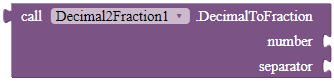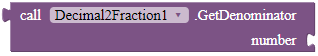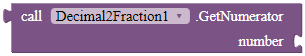# [F/OS] Decimal To Fraction Calculator! 🧮

Introduction

A simple extension that converts a decimal number to a fraction.

Version: 2

Blocks

DecimalToFractionConverts a decimal to a fraction.

Returns: text

Parameters: number = number (double), separator = text

GetDenominatorReturns the denominator of the fraction from the decimal.

Parameters: number = double

GetNumeratorReturns the numerator of the fraction from the decimal.

Parameters: number = double

Can this be done with built-in blocks?

Yes and no. As @ABG and I found out in this post, it really depends on what decimal you are converting. For some reason, AI2 floats decimals that are smaller than 0.00001. Hence, I recommend you to use this extension - you don't know what the user inputs.

TXT: Decimal2Fraction.txt (2.8 KB)

AIX: com.gordonlu.decimal2fraction.aix (6.4 KB)

Rate my extension!

• Good extension!

0 voters

KindlyPM me if you have any questions! Also, if you like my extension, pleaselike it! It takes some effort for me to make it...

Votes and likes tell me the general user feedback of my extension. If you read this extension, please take 20 seconds to drop by and give a vote / like!

If you have any features that you want to add and you know the code, PM me.

11 Likes

Simple, novel, effective1 Like

nice extension!

1 Like

Trouble logging in the extensions IDEthe source code will be posted ASAP.

its ok no problem @Gordon_Lu

take your time to upload1 Like

This extension is now Open Source. Free to modify. Thank you!

``````package com.gordonlu.decimal2fraction;

import android.app.Activity;
import android.content.Context;
import java.util.*;

@DesignerComponent(
version = 1,
description = "Converts a decimal into a fraction.",
category = ComponentCategory.EXTENSION,
nonVisible = true,

@SimpleObject(external = true)
//Libraries
@UsesLibraries(libraries = "")
//Permissions
@UsesPermissions(permissionNames = "")

public class Decimal2Fraction extends AndroidNonvisibleComponent {

//Activity and Context
private Context context;
private Activity activity;

public Decimal2Fraction(ComponentContainer container){
super(container.\$form());
this.activity = container.\$context();
this.context = container.\$context();
}

@SimpleFunction(description = "Turns a decimal into a fraction.")
public static String DecimalToFraction(double number, String separator) {
double intVal = Math.floor(number);
double fVal = number - intVal;
final long pVal = 1000000000;
long gcdVal = gcd(Math.round(fVal * pVal), pVal);

long num = Math.round(fVal * pVal) / gcdVal;
long deno = pVal / gcdVal;
return ((long)(intVal * deno) +
num + separator + deno);
}
@SimpleFunction(description = "Gets the numerator from the decimal.")
public static long GetNumerator(double number) {
double intVal = Math.floor(number);
double fVal = number - intVal;
final long pVal = 1000000000;
long gcdVal = gcd(Math.round(fVal * pVal), pVal);

long num = Math.round(fVal * pVal) / gcdVal;
long deno = pVal / gcdVal;
return ((long)(intVal * deno) +
num);
}
@SimpleFunction(description = "Gets the denominator from the decimal.")
public static long GetDenominator(double number) {
double intVal = Math.floor(number);
double fVal = number - intVal;
final long pVal = 1000000000;
long gcdVal = gcd(Math.round(fVal * pVal), pVal);

long num = Math.round(fVal * pVal) / gcdVal;
long deno = pVal / gcdVal;
return ((long)deno);
}
private static long gcd(long a, long b)
{
if (a == 0)
return b;
else if (b == 0)
return a;
if (a < b)
return gcd(a, b % a);
else
return gcd(b, a % b);
}
}
``````
2 Likes